• Python绘制频率分布直方图项目中在前期经常要看下数据的分布情况，这对于探究数据规律非常有用。概率分布表示样本数据的模样，长的好不好看如果有图像展示出来就非常完美了，使用Python绘制频率分布直方图非常简洁，...
Python绘制频率分布直方图项目中在前期经常要看下数据的分布情况，这对于探究数据规律非常有用。概率分布表示样本数据的模样，长的好不好看如果有图像展示出来就非常完美了，使用Python绘制频率分布直方图非常简洁，因为用的频次非常高，这里记录下来。还是Python大法好，代码简洁不拖沓~如果数据取值的范围跨度不大，可以使用等宽区间来展示直方图，这也是最常见的一种；如果数据取值范围比较野，也可以自定义区间端点，绘制图像，下面分两种情况展示plt.hist函数中有个参数normed默认是None，表示不对数据进行归一化，这个情况绘制出来的就是频次直方图，加了normed=True,之后就是标准的频率直方图1. 区间长度相同绘制直方图#-*- encoding=utf-8 -*-import datetimeimport numpy as npimport matplotlib.pyplot as pltimport matplotlibzhfont1 = matplotlib.font_manager.FontProperties(fname='C:\Windows\Fonts\simsun.ttc')# 按照固定区间长度绘制频率分布直方图# bins_interval 区间的长度# margin        设定的左边和右边空留的大小def probability_distribution(data, bins_interval=1, margin=1):bins = range(min(data), max(data) + bins_interval - 1, bins_interval)print(len(bins))for i in range(0, len(bins)):print(bins[i])plt.xlim(min(data) - margin, max(data) + margin)plt.title("Probability-distribution")plt.xlabel('Interval')plt.ylabel('Probability')# 频率分布normed=True，频次分布normed=Falseprob,left,rectangle = plt.hist(x=data, bins=bins, normed=True, histtype='bar', color=['r'])for x, y in zip(left, prob):# 字体上边文字# 频率分布数据 normed=Trueplt.text(x + bins_interval / 2, y + 0.003, '%.2f' % y, ha='center', va='top')# 频次分布数据 normed=False# plt.text(x + bins_interval / 2, y + 0.25, '%.2f' % y, ha='center', va='top')plt.show()2. 区间长度不同绘制频次直方图#-*- encoding=utf-8 -*-import datetimeimport numpy as npimport matplotlib.pyplot as pltimport matplotlibzhfont1 = matplotlib.font_manager.FontProperties(fname='C:\Windows\Fonts\simsun.ttc'# 自己给定区间，小于区间左端点和大于区间右端点的统一做处理，对于数据分布不均很的情况处理较友好# bins      自己设定的区间数值列表# margin    设定的左边和右边空留的大小# label     右上方显示的图例文字"""eimport numpy as npdata = np.random.normal(0, 1, 1000)bins = np.arange(-5, 5, 0.1)probability_distribution_extend(data=data, bins=bins)"""def probability_distribution_extend(data, bins, margin=1, label='Distribution'):bins = sorted(bins)length = len(bins)intervals = np.zeros(length+1)for value in data:i = 0while i < length and value >= bins[i]:i += 1intervals[i] += 1intervals = intervals / float(len(data))plt.xlim(min(bins) - margin, max(bins) + margin)bins.insert(0, -999)plt.title("probability-distribution")plt.xlabel('Interval')plt.ylabel('Probability')plt.bar(bins, intervals, color=['r'], label=label)plt.legend()plt.show()Case示例if __name__ == '__main__':data = [1,4,6,7,8,9,11,11,12,12,13,13,16,17,18,22,25]probability_distribution(data=data, bins_interval=5,margin=0)效果如下图频次分布直方图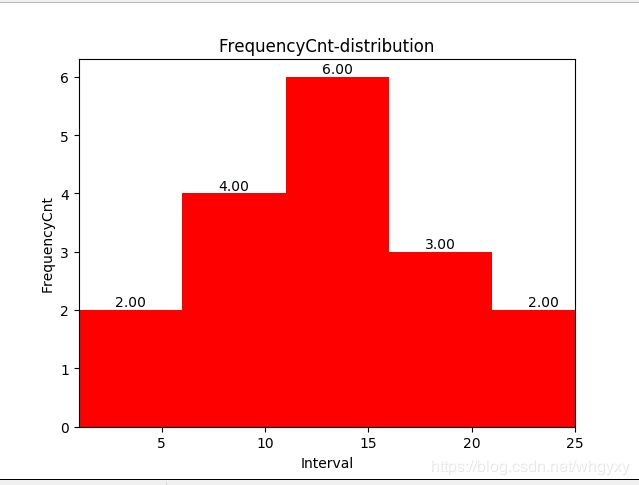频率分布直方图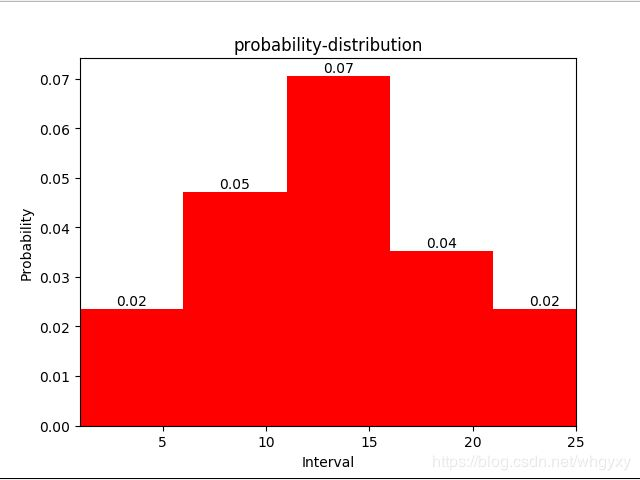展开全文• 现在人工智能越来越实用，甚至深入到千家万户，随之而来的就是python技术的火爆，今天小猿圈python讲师为你讲解一下绘制频率分布直方图示例，希望对于刚刚自学python的你有一定的帮助。项目中在前期经常要看下数据的...
现在人工智能越来越实用，甚至深入到千家万户，随之而来的就是python技术的火爆，今天小猿圈python讲师为你讲解一下绘制频率分布直方图示例，希望对于刚刚自学python的你有一定的帮助。项目中在前期经常要看下数据的分布情况，这对于探究数据规律非常有用。概率分布表示样本数据的模样，长的好不好看如果有图像展示出来就非常完美了，使用Python绘制频率分布直方图非常简洁，因为用的频次非常高，这里记录下来。还是Python大法好，代码简洁不拖沓~如果数据取值的范围跨度不大，可以使用等宽区间来展示直方图，这也是最常见的一种；如果数据取值范围比较野，也可以自定义区间端点，绘制图像，下面分两种情况展示1.区间长度相同绘制直方图#-*- encoding=utf-8 -*-import datetimeimport numpy as npimport matplotlib.pyplot as pltimport matplotlibzhfont1 = matplotlib.font_manager.FontProperties(fname='C:WindowsFontssimsun.ttc')# 按照固定区间长度绘制频率分布直方图# bins_interval 区间的长度# margin    设定的左边和右边空留的大小def probability_distribution(data, bins_interval=1, margin=1):  bins = range(min(data), max(data) + bins_interval - 1, bins_interval)  print(len(bins))  for i in range(0, len(bins)):    print(bins[i])  plt.xlim(min(data) - margin, max(data) + margin)  plt.title("probability-distribution")  plt.xlabel('Interval')  plt.ylabel('Probability')  plt.hist(x=data, bins=bins, histtype='bar', color=['r'])  plt.show()2.区间长度不同绘制直方图#-*- encoding=utf-8 -*-import datetimeimport numpy as npimport matplotlib.pyplot as pltimport matplotlibzhfont1 = matplotlib.font_manager.FontProperties(fname='C:WindowsFontssimsun.ttc'# 自己给定区间，小于区间左端点和大于区间右端点的统一做处理，对于数据分布不均很的情况处理较友好# bins  自己设定的区间数值列表# margin  设定的左边和右边空留的大小# label  右上方显示的图例文字"""e  import numpy as np  data = np.random.normal(0, 1, 1000)  bins = np.arange(-5, 5, 0.1)  probability_distribution_extend(data=data, bins=bins)"""def probability_distribution_extend(data, bins, margin=1, label='Distribution'):  bins = sorted(bins)  length = len(bins)  intervals = np.zeros(length+1)  for value in data:    i = 0    while i < length and value >= bins[i]:      i += 1    intervals[i] += 1  intervals = intervals / float(len(data))  plt.xlim(min(bins) - margin, max(bins) + margin)  bins.insert(0, -999)  plt.title("probability-distribution")  plt.xlabel('Interval')  plt.ylabel('Probability')  plt.bar(bins, intervals, color=['r'], label=label)  plt.legend()  plt.show()Case示例if __name__ == '__main__':  data = [1,4,6,7,8,9,11,11,12,12,13,13,16,17,18,22,25]  probability_distribution(data=data, bins_interval=5,margin=0)以上就是小猿圈python讲师给大家分享的绘制频率分布直方图示例，希望对小伙伴们有所帮助，想要了解更多内容的小伙伴可以到小猿圈直接观看，想要学好Python开发技术的小伙伴快快行动吧。
展开全文• 概率分布表示样本数据的模样，长的好不好看如果有图像展示出来就非常完美了，使用Python绘制频率分布直方图非常简洁，因为用的频次非常高，这里记录下来。还是Python大法好，代码简洁不拖沓~如果数据取值的范围跨度...
项目中在前期经常要看下数据的分布情况，这对于探究数据规律非常有用。概率分布表示样本数据的模样，长的好不好看如果有图像展示出来就非常完美了，使用Python绘制频率分布直方图非常简洁，因为用的频次非常高，这里记录下来。还是Python大法好，代码简洁不拖沓~如果数据取值的范围跨度不大，可以使用等宽区间来展示直方图，这也是最常见的一种；如果数据取值范围比较野，也可以自定义区间端点，绘制图像，下面分两种情况展示1. 区间长度相同绘制直方图#-*- encoding=utf-8 -*-import datetimeimport numpy as npimport matplotlib.pyplot as pltimport matplotlibzhfont1 = matplotlib.font_manager.FontProperties(fname='C:\Windows\Fonts\simsun.ttc')# 按照固定区间长度绘制频率分布直方图# bins_interval 区间的长度# margin    设定的左边和右边空留的大小def probability_distribution(data, bins_interval=1, margin=1):bins = range(min(data), max(data) + bins_interval - 1, bins_interval)print(len(bins))for i in range(0, len(bins)):print(bins[i])plt.xlim(min(data) - margin, max(data) + margin)plt.title("probability-distribution")plt.xlabel('Interval')plt.ylabel('Probability')plt.hist(x=data, bins=bins, histtype='bar', color=['r'])plt.show()2. 区间长度不同绘制直方图#-*- encoding=utf-8 -*-import datetimeimport numpy as npimport matplotlib.pyplot as pltimport matplotlibzhfont1 = matplotlib.font_manager.FontProperties(fname='C:\Windows\Fonts\simsun.ttc'# 自己给定区间，小于区间左端点和大于区间右端点的统一做处理，对于数据分布不均很的情况处理较友好# bins   自己设定的区间数值列表# margin  设定的左边和右边空留的大小# label   右上方显示的图例文字"""eimport numpy as npdata = np.random.normal(0, 1, 1000)bins = np.arange(-5, 5, 0.1)probability_distribution_extend(data=data, bins=bins)"""def probability_distribution_extend(data, bins, margin=1, label='Distribution'):bins = sorted(bins)length = len(bins)intervals = np.zeros(length+1)for value in data:i = 0while i < length and value >= bins[i]:i += 1intervals[i] += 1intervals = intervals / float(len(data))plt.xlim(min(bins) - margin, max(bins) + margin)bins.insert(0, -999)plt.title("probability-distribution")plt.xlabel('Interval')plt.ylabel('Probability')plt.bar(bins, intervals, color=['r'], label=label)plt.legend()plt.show()Case示例if __name__ == '__main__':data = [1,4,6,7,8,9,11,11,12,12,13,13,16,17,18,22,25]probability_distribution(data=data, bins_interval=5,margin=0)效果如下图以上这篇Python绘制频率分布直方图的示例就是小编分享给大家的全部内容了，希望能给大家一个参考，也希望大家多多支持脚本之家。
展开全文• 前言最近接到一个任务，来绘制一组数据的频率分布直方图。直方图是高中就学习过的知识，但是在科研、数据分析中仍然占有很高的地位。一开始我认为这个只需要用Excel就可以轻松实现，但是对Excel的绘图不熟悉，折腾了...
前言最近接到一个任务，来绘制一组数据的频率分布直方图。直方图是高中就学习过的知识，但是在科研、数据分析中仍然占有很高的地位。一开始我认为这个只需要用Excel就可以轻松实现，但是对Excel的绘图不熟悉，折腾了半天才用Python实现。本次实验中对数据预处理和绘图的经历有一定的借鉴意义。因此记录下来。数据预处理不同于其他插图，直方图只需要一列数据即可。表示的是这一列数据中各个数据出现的频率分布。实验用的数据是微博数据，经过处理后得到了一系列微博用户的轨迹数据。如图sum2是轨迹的数量，line是轨迹编号。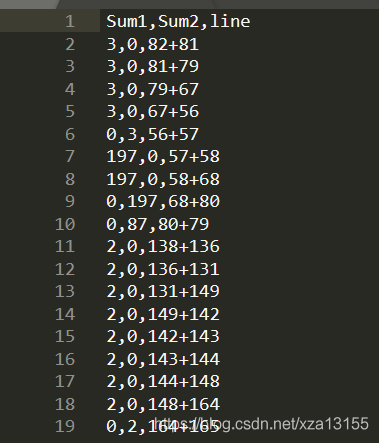因为是不同用户的轨迹，因此有重复需要通过arcgis的汇总功能，以line为key，对sum2求和。Python的group_by函数也可以实现这个功能。最终得到了轨迹的数量；绘制轨迹数的直方图，能够分析研究区域内轨迹被用户访问的频率。如果有一些访问频率过低的轨迹，那就属于无效数据，需要进行区分。绘制直方图1，Excel绘制数据导出为excel表格格式。选中一列数据，然后插入直方图。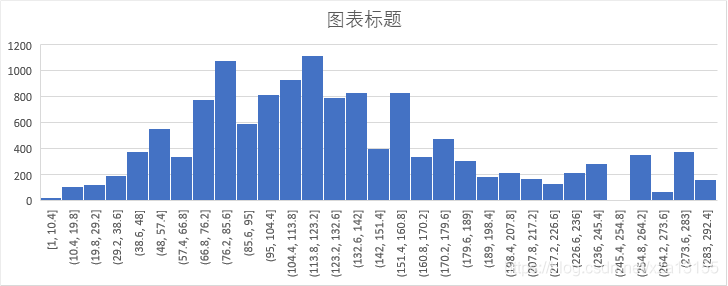初始化的图下标不是很合适。可以点击x轴，选择“设置坐标轴”菜单。在箱宽度中可以设置箱的宽度，即直方图的组距。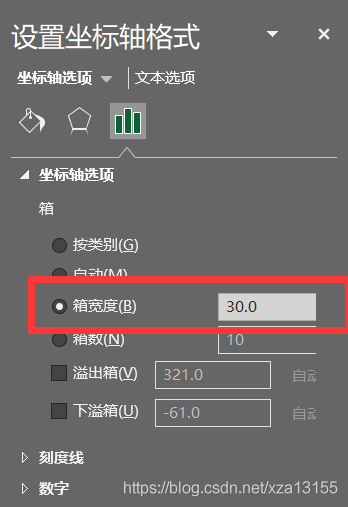下图为调好的图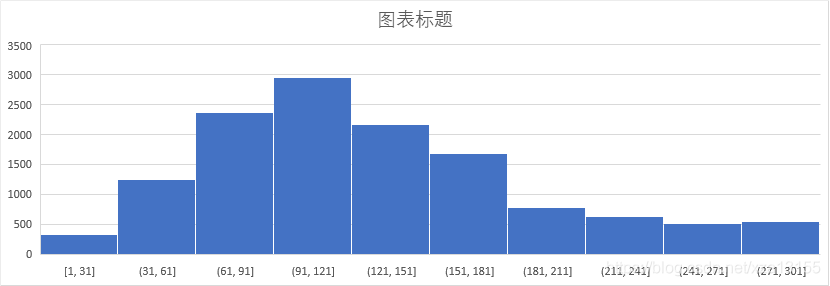2，Python实现Python则可以利用matplotlib库实现绘制。下面附上代码；其中a为list元素。如a=[1,2,3,4,5,6,7]from matplotlib import pyplot as plt# 计算组数d = 800 # 组距num_bins = int((max(a) - min(a)) // d)print(max(a), min(a), max(a) - min(a))print(num_bins)# 设置图形大小plt.figure(figsize=(20, 13), dpi=80)# 绘制直方图plt.hist(a, num_bins)# 设置x轴的刻度plt.xticks(range(int(min(a)), int(max(a)) + d, d))plt.grid()plt.show()此外在使用Python时遇到了一个问题，去除list中值为0的元素。如果使用for循环遍历，则可能出现去除残余的现象，这是因为每次用remove删除值为0的元素时，list的下一个元素就会前移，而指针向后移导致遗漏。for i in range(a):if(i==0):a.remove(i)正确的方法如下：import pandas as pddf=pd.read_csv("游客数据.csv",encoding='utf-8')    #读取date文件a=df['FREQUENCY'].tolist()while 0 in a:a.remove(0)原文链接:https://blog.csdn.net/xza13155/article/details/113822439
展开全文• 概率分布表示样本数据的模样，长的好不好看如果有图像展示出来就非常完美了，使用Python绘制频率分布直方图非常简洁，因为用的频次非常高，这里记录下来。还是Python大法好，代码简洁不拖沓~如果数据取值的范围跨度...
• 概率分布表示样本数据的模样，长的好不好看如果有图像展示出来就非常完美了，使用Python绘制频率分布直方图非常简洁，因为用的频次非常高，这里记录下来。还是Python大法好，代码简洁不拖沓~如果数据取值的范围跨度...
• 现在人工智能越来越实用，甚至深入到千家万户，随之而来的就是python技术的火爆，今天小猿圈python讲师为你讲解一下绘制频率分布直方图示例，希望对于刚刚自学python的你有一定的帮助。项目中在前期经常要看下数据的...
• Python画频率分布直方图，不显示图形怎么办Python画频率分布直方图导入数据并作直方图图形不显示的解决方法 Python画频率分布直方图 我们在拿到一组数据时，为了观察其大致分布，常常需要绘制其频率分布直方图。下面...python 数据分析
• Python绘制频率分布直方图 项目中在前期经常要看下数据的分布情况，这对于探究数据规律非常有用。概率分布表示样本数据的模样，长的好不好看如果有图像展示出来就非常完美了，使用Python绘制频率分布直方图非常简洁...
• #画频率分布直方图 #纵坐标名字为frequency，频数直方图。则使用：hist(x,freq=T) #纵坐标名字为density，频率直方图。则使用：hist(x,freq=F) hist(audit1[,1],col='white',freq=T)r语言 频数分布直方图
• 今天小编就为大家分享一篇Python绘制频率分布直方图的示例，具有很好的参考价值，希望对大家有所帮助。一起跟随小编过来看看吧
• 在数理统计中，会经常使用到频率分布直方图，能够直观的反应频率分布的范围大小，在直角坐标系中，横轴为统计变量取值，横轴上的每个小区间对应一个组的组距，作为小矩形的底边；纵轴表示频率与组距的比值，并用它作...
• 这类题目一般出现在大题，要求同学们对于如何分组，频率分布直方图和频率分布表有正确的认识和理解相关的含义， (1)频数：每个小组内的数据的个数叫做该组的频数。各个小组的频数之和等于数据总数n。 (2)频率：每个...
• 在这里是以分组边界值为“X”来计算：Mean=AVERAGE(A:A)(数据算术平均)Standard_dev=STDEV(A:A)(数据的标准方差)Cumulative=0(概率密度函数)道向下填充在直方图中增加正态分专布曲线图1、在直方图内右键→选择数据→...
• 频率分布直方图是频率分布表的图形表示，可以帮助研究者发现某些离群值或极端值，更好地揭示变量的分布类型(对称分布、正偏锋分布、负偏峰分布)与分布特征(集中趋势、离散趋势)，为研究者进一步计算统计指标和统计...
• 直方图能帮助迅速了解数据的分布形态，将观测数据分组，并以柱状条表示各分组中观测数据的个数。简单而有效的可视化方法，可检测数据是否有问题，也可看出数据是否遵从某种已知分布。本次案例通过生成深圳市疫情个案...
• 茎叶 知识回忆 初中统计部分曾学过用平均数、众数、中位数反映总体的集中水平 1、众 数：在一组数据中，出现次数最多的数据叫做这组数据的众数； 2、中位数：将一组数据按大小依次排列，把处在最中间位置的一个...
• 一、什么是直方图 1、定义 直方图是一种条形图，是以组距为底边、以频数为高度的一系列连接起来的直方型矩形图 2、相关概念 组数：在统计数据时，把数据按照不同的范围分成几个组，分成的组的个数称为组数。 ...数据分析 Excel
• % 画频率分布直方图 [counts,centers] = hist(x, 7); figure bar(centers, counts / sum(counts)) % 分布参数拟合 [mu,sigma]=normfit(x); % 画已知分布的概率密度曲线 x1 = -4:0.1:4; y1 = pdf('...Matlab
• 这篇经验告诉你，如何利用python的matplotlib模块画直方图。工具/原料windows系统电脑一台python软件，并安装matplotlib模块spyder编辑器方法/步骤1第一步，点击键盘 win+r，打开运行窗口；在窗口中输入“cmd"，点击...
• 频数分布直方图能清楚地显示各组频数分布情况，同时直观展示各组之间频数的差别，是数据分析过程中常用的一种图。画频数分布直方图的方法有很多，可以用SPSS、Excel等等，但这些工具操作起来比较繁琐，在这里小编教...
• 语音非均匀量化前后的输出电平频率分布直方图对比GUI。采样率、bit位数可通过滑块调节，压缩方案通过菜单选择，至少要包括A率以及u率压缩。
• 现在人工智能越来越实用，甚至深入到千家万户，随之而来的就是python技术的火爆，今天小猿圈python讲师为你讲解一下绘制频率分布直方图示例，希望对于刚刚自学python的你有一定的帮助。项目中在前期经常要看下数据的...
• 直方图是用来描述数据分布的统计报告图，体现了数据分布在某个区间或者取得某个值的频数或频率，如果在统计数据时将区间划分的足够小，则频率分布直方图就会变成一条平滑曲线，即为密度图，事实上，我们常常提及的......